## ROPE ELEMENTS WITH MOVING NODES IN ROPE-PULLEY SYSTEMS 1)

Qi Zhaohui,2), Guo Shudong, Zhuo Yingpeng

Department of Engineering Mechanics, Dalian University of Technology, Dalian 116024, China

 基金资助: 1) 国家自然科学基金资助项目.  118721371) 国家自然科学基金资助项目.  91748203

Received: 2019-06-29   Accepted: 2019-09-20   Online: 2019-09-20Abstract

In traditional finite element methods, a common hypothesis is the nodes of an element being fixed with material points, which place restrictions on the description of a system. In this paper, this hypothesis is released and a new kind of rope element with nodes being no longer fixed with either spatial coordinates or material coordinates is presented. The theory of obtaining material velocity and acceleration of a point from the corresponding spatial velocity and acceleration of the point is established. The velocities and accelerations in the principle of virtual power should being material velocities and accelerations respectively is emphasized and applied. According to the feature of a rope-pulley system, its movement can be described by a new group of variables, such as arc-lengths, azimuthal angles and strains of ropes at entrance and exit contacting points. Instead of traditional methods, conditions of contact between rope and pulley are modeled as the material velocity of a point on the contacting rope being equal to the corresponding point on the pulley. By means of the presented methods, some obstacles, such as frequently divergence and high time consuming that resulting from traditional finite element methods can be removed. Motion equation of the rope-pulley system is derived by the principle of virtual power. Arc-length coordinates, positions of contact boundary points, the movement of pulley centers and their body-fixed reference coordinate systems, shape and positions changing of ropes as well as the tension forces in every point of a rope, can be obtained high precisely. The presented method can provide a new theoretical basis for analysis of mechanical systems with ropes and pulleys. The presented theory of using spatial points as describing variables to replace traditional material points is of applicable widely. It can be a reference for theory and application of finite element methods.

Keywords： dynamics of multi-flexible bodies ; finite element methods ; rope-pulley system ; moving node ; spatial description

Qi Zhaohui, Guo Shudong, Zhuo Yingpeng. ROPE ELEMENTS WITH MOVING NODES IN ROPE-PULLEY SYSTEMS 1). Chinese Journal of Theoretical and Applied Mechanics[J], 2019, 51(6): 1856-1871 DOI:10.6052/0459-1879-19-168

## 引言

2011年, 文献为研究物体在一维介质中的运动提出了任意欧拉$\!-\!$拉格朗日绝对节点坐标单元(ALE-ANCF). 这类单元解除了单元节点与物质点的绑定, 并很快在求解一些工程问题中得到了应用[33-35]. 充分说明了采用空间点描述系统运动的必要性和可能性.

## 1 物质速度和空间速度

$\begin{eqnarray}{{\dot{ r}}} = \frac{\partial {{ r}}}{\partial t} +\frac{\partial {{ r}}}{\partial s}\dot{s}\end{eqnarray}$

### 图1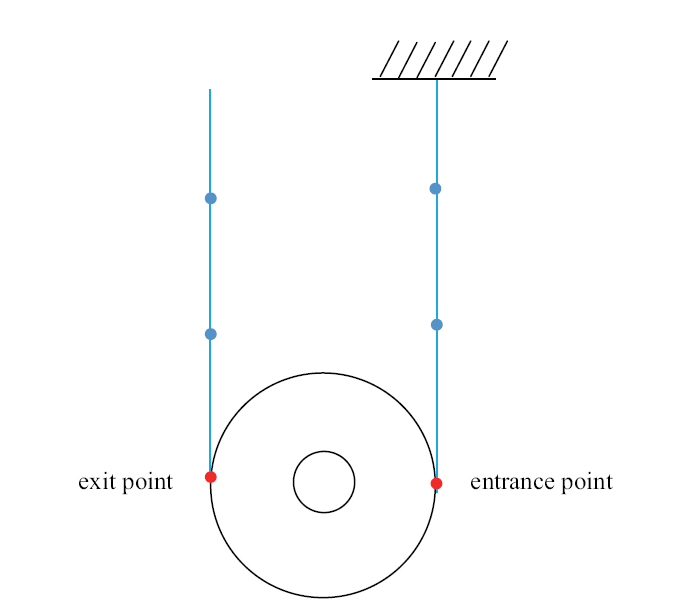Fig.1   An example of spatial points: Boundary points of a rope-pulley system

$\begin{eqnarray}{{\ddot{ r}}} = \frac{\partial ^2{{ r}}}{\partial t^2} +2\frac{\partial ^2{{ r}}}{\partial s\partial t}\dot{s} +\frac{\partial ^2{{ r}}}{\partial s^2}\dot{s}^2 + \frac{\partial{{ r}}}{\partial s}\ddot{s}\end{eqnarray}$

## 2 虚功率原理

$\begin{eqnarray}&&\int_0^L {\delta \left( {{\partial {{ r}}}/{\partial t}} \right)^{\rm T}{\partial ^2{{ r}}}/{\partial t^2}\rho {\rm d}s} + \\&&\qquad\int_0^L{\delta \left( {{\partial {{ \varepsilon }}}/{\partial t}} \right)^{\rm T}{{ \sigma }}{\rm d}s} =\delta p_f\end{eqnarray}$

$\begin{eqnarray}\varepsilon = \| {{ {r}'}} \| - 1\end{eqnarray}$

$\begin{eqnarray}\sigma = E_A \varepsilon\end{eqnarray}$

$\begin{eqnarray}&& \bar{\sigma } = \frac{1}{h}\int_0^h {\left( {\sigma + \tau{\partial \sigma }/{\partial t} + \frac12\tau ^2{\partial ^2\sigma }/{\partial t^2}}\right){\rm d}\tau } =\\&&\qquad E_A \left( {\varepsilon +\frac12h{\partial\varepsilon }/{\partial t} +\frac16h^2{\partial ^2\varepsilon }/{\partial t^2}} \right)\end{eqnarray}$

$\begin{eqnarray}&&{\partial \varepsilon }/{\partial t} = \dot{\varepsilon } - {\varepsilon }'\dot{s} \end{eqnarray}$
$\begin{eqnarray}&&{\partial ^2\varepsilon }/{\partial t^2} = \ddot{\varepsilon } + {\varepsilon }''\dot{s}^2 - {\varepsilon }'\ddot{s} - 2{\dot{\varepsilon }}'\dot{s}\end{eqnarray}$

$\begin{eqnarray}&&\dot{\varepsilon } = ( {1 + \varepsilon } )^{ - 1}( {{ {r}'}} )^{\rm T}{{\dot{ {r}'}}}\end{eqnarray}$
$\begin{eqnarray}&&\ddot{\varepsilon } = ( {1 + \varepsilon } )^{ - 1}\lt[{( {{ {r}'}} )^{\rm T}{{\ddot{ {r}'}}} + ( {{\dot{ {r}'}}} )^{\rm T}{{\dot{ {r}'}}} - \dot{\varepsilon }^2}]\end{eqnarray}$

$\varepsilon$的物质速度虚变分

$\begin{eqnarray}\delta \left( {{\partial \varepsilon }/{\partial t}} \right) = \left( {1 + \varepsilon } \right)^{ - 1}\left( {{ {r}'}} \right)^{\rm T}\delta {{\dot{ {r}'}}} - {\varepsilon }'\delta \dot{s}\end{eqnarray}$

$\begin{eqnarray}\delta \left( {{\partial {{ r}}}/{\partial t}} \right) = \delta {{\dot{ r}}} - {{ {r}'}}\delta \dot{s}\end{eqnarray}$

## 3 滑轮间绳索单元

### 图2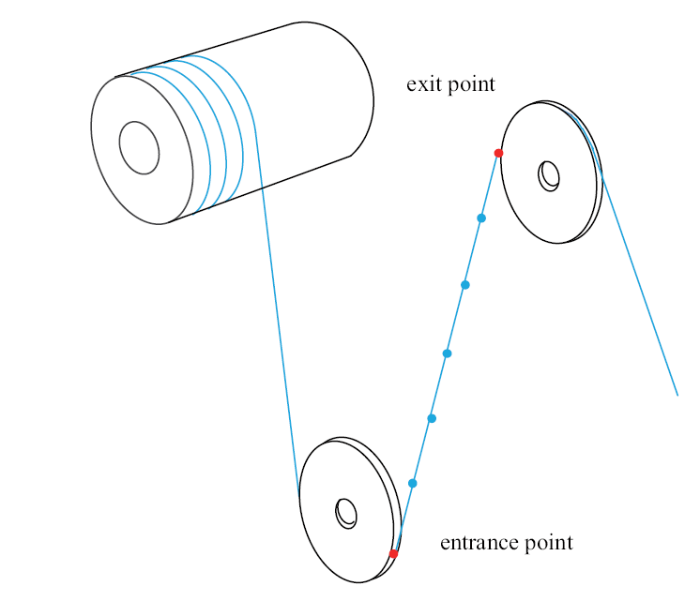Fig.2   A rope element between two pulleys

$\begin{eqnarray}s_i = n^{ - 1}\left( {n - i} \right)s_0 + n^{ - 1}is_n\end{eqnarray}$

$\begin{eqnarray}{{ r}} = N_1 {{ r}}_i + N_2 {{ r}}_{i + 1} + L(N_3 {{ {r}'}}_i + N_4 {{ {r}'}}_{i + 1} )\end{eqnarray}$

$\begin{eqnarray}&&N_1 = 1 - 3\xi ^2 + 2\xi ^3 \end{eqnarray}$
$\begin{eqnarray}&&N_2 = 3\xi ^2 - 2\xi ^3 \end{eqnarray}$
$\begin{eqnarray}&&N_3 = \xi - 2\xi ^2 + \xi ^3 \end{eqnarray}$
$\begin{eqnarray}&&N_4 = \xi ^3 - \xi ^2 \end{eqnarray}$

$\begin{eqnarray}\xi = L^{ - 1}( {s - s_i })\end{eqnarray}$

$\begin{eqnarray}\dot{L} = n^{ - 1}\left( {\dot{s}_n - \dot{s}_0 } \right) \buildrel \Delta \over = {{ T}}_L {{\dot{ q}}}_r \end{eqnarray}$

$\begin{eqnarray}\dot{s}_i = n^{ - 1}\left( {n - i} \right)\dot{s}_0 + n^{ - 1}i\dot{s}_n\end{eqnarray}$

$\begin{eqnarray}\dot{s} = \dot{L}\xi + \dot{s}_i \buildrel \Delta \over = {{ T}}_s{{\dot{ q}}}_r\end{eqnarray}$

$\begin{eqnarray}[ {{ {r}'}} ]{{ A}} = a[ {{ r}}]{{ B}} + [ {{{ {r}'}}_0 } \ \ {{ {r}'}}_n]{{ C}}\end{eqnarray}$

$\begin{eqnarray}&&[ {{\dot{ {r}'}}} ] = ( {a[ {{\dot{ r}}} ] + \dot{a}[ {{ r}} ]} ){{ B}}_a+ [ {{\begin{array}{*{20}c} {{{\dot{ {r}'}}}_0 } \ \ {{{\dot{ {r}'}}}_n } {{ C}}_a \end{array} }} ]\end{eqnarray}$
$\begin{eqnarray}&&[ {{\ddot{ {r}'}}} ] = ( {a[ {{\ddot{ r}}} ] + 2\dot{a}[ {{\dot{ r}}} ] + \ddot{a}[ {{ r}} ]} ){{ B}}_a + [{{ {{{\ddot{ {r}'}}}_0 } \ \ {{{\ddot{ {r}'}}}_n } \\ }} ]{{ C}}_a\end{eqnarray}$

$\begin{eqnarray}{{\dot{ {r}'}}}_i = ( {[ {{\begin{array}{*{20}c} {a{{ Y}}_i } \ \ {{{ \varGamma }}_i } \ \ { 0_{3\times2} } \\\end{array} }} ] - a^2[ {{ r}} ]{{ B}}_a {{ T}}_L } ){{\dot{ q}}}_r \buildrel \Delta \over = {{ \varPhi }}_i {{\dot{ q}}}_r\end{eqnarray}$

${{ Y}}_i = [ {{{b_{1,i} {{ E}}} \ \ {b_{2,i} {{ E}}} \ \ \cdots\ \ {b_{n + 1,i} {{ E}}} }} ]$
${{\varGamma}}_i = [ {c_{1,i} {{ E}}} {c_{2,i} {{ E}}}]$

$E$为$3\times 3$维单位矩阵. 式(25)可以改写为

$\begin{eqnarray}{{\ddot{ {r}'}}}_i = {{ \varPhi }}_i {{\ddot{ q}}}_r +{{ \gamma }}_i\end{eqnarray}$

$\begin{eqnarray}{{ \gamma }}_i = - 2a^2\dot{L}\sum_{k = 0}^n {b_{k + 1,i}{{\dot{ r}}}_k } + 2a^3\dot{L}^2\sum_{k = 0}^n {b_{k + 1,i}{{ r}}_k }\end{eqnarray}$

$\begin{eqnarray}&&{{ {r}'}} = a( {{N}'_1 {{ r}}_i + {N}'_2 {{ r}}_{i + 1} } ) + {N}'_3 {{ {r}'}}_i + {N}'_4 {{ {r}'}}_{i + 1}\\\end{eqnarray}$
$\begin{eqnarray}&&{{ {r}''}} = a^2({N}''_1 \,{{ r}}_i + {N}''_2 \,{{ r}}_{i + 1} ) + a( {{N}''_3 \,{{ {r}'}}_i + {N}''_4 \,{{ {r}'}}_{i + 1} } )\\\end{eqnarray}$
$\begin{eqnarray}&&{{ {r}'''}} = a^3( {{N}'''_1 \,{{ r}}_i + {N}'''_2 \,{{ r}}_{i + 1} } ) + a^2({N}'''_3 \,{{ {r}'}}_i + {N}'''_4\,{{ {r}'}}_{i + 1} )\qquad\end{eqnarray}$

$\begin{eqnarray} {{\dot{ {r}}}} = {{\bar{ T}}}_{r} {{\dot{ q}}}_r,\ \ {{\ddot{ {r}}}} = {{\bar{ T}}}_{r} {{\ddot{ q}}}_r+ {{\bar{ \alpha }}}_{r} \end{eqnarray}$
$\begin{eqnarray} {{\dot{ {r}'}}} = {{\bar{ T}}}_{r}' {{\dot{ q}}}_r,\ \ {{\ddot{ {r}'}}} = {{\bar{ T}}}_{r}' {{\ddot{ q}}}_r+ {{\bar{ \alpha }}}_{r}' \end{eqnarray}$

$\begin{eqnarray}&&{\bar{ T}}_r = N_1 {{ G}}_i + N_2 {{ G}}_{i + 1} + L(N_3{{ \varPhi }}_i + N_4 {{ \varPhi }}_{i + 1} )+\\&&\qquad(N_3 {{ {r}'}}_i + N_4 {{ {r}'}}_{i + 1} ){{ T}}_L \end{eqnarray}$
$\begin{eqnarray}&&{\bar{ T}}_{r}' = a( {{N}'_1 {{ G}}_i + {N}'_2 {{ G}}_{i + 1} } ) + {N}'_3 {{ \varPhi }}_i + {N}'_4 {{ \varPhi}}_{i + 1} -\\&&\qquad a^2( {{N}'_1 {{ r}}_i + {N}'_2 {{ r}}_{i + 1} } ){{ T}}_L\end{eqnarray}$

$\begin{eqnarray}&&{\bar{ \alpha }}_r = L(N_3 {{ \gamma }}_i + N_4 {{ \gamma}}_{i + 1} ) + 2\dot{L}(N_3 {{\dot{ {r}'}}}_i + N_4 {{\dot{ {r}'}}}_{i + 1} ) \end{eqnarray}$
$\begin{eqnarray}&&{\bar{ \alpha }}_{r}' = {N}'_3 {{ \gamma }}_i + {N}'_4 {{ \gamma }}_{i + 1} \mbox{ + 2}\dot{a}( {{N}'_1 {{\dot{ r}}}_i +{N}'_2 {{\dot{ r}}}_{i + 1} } ) +\\&&\qquad 2a^3\dot{L}^2({{N}'_1 {{ r}}_i + {N}'_2 {{ r}}_{i + 1} } )\end{eqnarray}$

$\begin{eqnarray}&&{\partial {{ r}}}/{\partial t} = {{\dot{ r}}} - {{ {r}'}}\dot{s} \buildrel \Delta \over = {{ T}}_r {{\dot{ q}}}_r\end{eqnarray}$
$\begin{eqnarray}&&{\partial ^2{{ r}}}/{\partial t^2} ={{\ddot{ r}}} - {{ {r}'}}\ddot{s} - 2{{\dot{ {r}'}}}\dot{s} + {{ {r}''}}\dot{s}^2 = {{ T}}_r {{\ddot{ q}}}_r + {{ a}}_r\end{eqnarray}$

$\begin{eqnarray}{{ T}}_r = {{\bar{ T}}}_r - {{ {r}'T}}_s\end{eqnarray}$

$\begin{eqnarray}{{ a}}_r = {{\bar{ a}}}_r + {{ {r}''}}\dot{s}^2 -2{{\dot{ {r}'}}}\dot{s}\end{eqnarray}$

$\begin{eqnarray}\delta p_m = \int_0^L {\delta ( {{\partial {{ r}}}/{\partial t}} )^{\rm T}{\partial ^2{{ r}}}/{\partial t^2}\rho {\rm d}s} = \delta {{\dot{ q}}}^{\rm T}_r ( {{{ M}}_r {{\ddot{ q}}}_r + {{ f}}_r } )\quad \end{eqnarray}$

$\begin{eqnarray}{{ M}}_r = \rho L\int_0^1 {{{ T}}_r^{\rm T} {{ T}}_r{\rm d}\xi }\end{eqnarray}$

$\begin{eqnarray}{{ f}}_r = \rho L\int_0^1 {{{ T}}_r^{\rm T} {{ a}}_r{\rm d}\xi }\end{eqnarray}$

$\begin{eqnarray}\dot{\varepsilon } = {{\bar{ T}}}_\varepsilon {{\dot{ q}}}_r,\ddot{\varepsilon } = {{\bar{ T}}}_\varepsilon {{\ddot{ q}}}_r + \bar{\alpha }_\varepsilon \end{eqnarray}$

$\begin{eqnarray}{{\bar{ T}}}_\varepsilon = ( {1 + \varepsilon } )^{ -1}( {{ {r}'}} )^{\rm T}{{\bar{ T}}}_{r}'\end{eqnarray}$

$\begin{eqnarray}\bar{\alpha }_\varepsilon = ( {1 + \varepsilon } )^{ - 1}\lt[{( {{\dot{ {r}'}}} )^{\rm T}{{\dot{ {r}'}}} + ({{ {r}'}} )^{\rm T}{{\bar{ \alpha }}}_{r}' - \dot{\varepsilon }^2} ]\end{eqnarray}$

$\begin{eqnarray}&&{\varepsilon }' = ( {1 + \varepsilon } )^{ - 1}( {{ {r}'}} )^{\rm T}{{ {r}''}}\end{eqnarray}$
$\begin{eqnarray}&&{\varepsilon }'' = ( {1 + \varepsilon } )^{ - 1}[ {({{ {r}''}} )^{\rm T}{{ {r}''}} + ( {{ {r}'}})^{\rm T}{{ {r}'''}} - ( {\varepsilon }' )^2}]\end{eqnarray}$

$\begin{eqnarray}\dot{{\varepsilon }'} = ( {1 + \varepsilon } )^{ - 1}[{( {{\dot{ {r}'}}} )^{\rm T}{{ {r}''}} + ( {{ {r}'}} )^{\rm T}{{\dot{ {r}''}}} - \dot{\varepsilon}{\varepsilon }'}]\end{eqnarray}$

$\begin{eqnarray}&&{\partial \varepsilon }/{\partial t} = {{ T}}_\varepsilon {{\dot{ q}}}_r \end{eqnarray}$
$\begin{eqnarray} &&{\partial ^2\varepsilon }/{\partial t^2} = {{ T}}_\varepsilon {{\ddot{ q}}}_r + \alpha_\varepsilon\end{eqnarray}$

$\begin{eqnarray}{{ T}}_\varepsilon = {{\bar{ T}}}_\varepsilon - {\varepsilon}'{{ T}}_s\end{eqnarray}$

$\begin{eqnarray}\alpha _\varepsilon = \bar{\alpha }_\varepsilon + {\varepsilon }''\dot{s}^2 - 2\dot{{\varepsilon }'}\dot{s}\end{eqnarray}$

$\begin{eqnarray}\delta p_\varepsilon = \int_0^L {\delta ( {{\partial \varepsilon}/{\partial t}} )^{\rm T}\bar{\sigma }{\rm d}s} = \delta{{\dot{ q}}}^{\rm T}_r ( {{{ M}}_\varepsilon {{\ddot{ q}}}_r + {{ f}}_\varepsilon } )\end{eqnarray}$

$\begin{eqnarray}{{ M}}_\varepsilon = \frac16h^2E_A L\int_0^1{{{ T}}_\varepsilon ^{\rm T} {{ T}}_\varepsilon {\rm d}\xi }\end{eqnarray}$

$\begin{eqnarray}{{ f}}_\varepsilon = E_A L\int_0^1 {{{ T}}_\varepsilon^{\rm T} \lt( {\varepsilon + \frac12h{\partial \varepsilon }/{\partial t} + \frac16h^2\alpha_\varepsilon } ){\rm d}\xi }\end{eqnarray}$

## 4 滑轮单元

$\begin{eqnarray}&&{{\bar{ e}}}_x = \cos \beta _2 {{ g}}_1 + \sin \beta _1 \sin \beta _2 {{ g}}_2 - \cos \beta _1 \sin \beta _2 {{ g}}_3\quad\end{eqnarray}$
$\begin{eqnarray}&&{{\bar{ e}}}_y = \cos \beta _1 {{ g}}_2 + \sin \beta _1 {{ g}}_3\end{eqnarray}$
$\begin{eqnarray}&&{{\bar{ e}}}_z = \sin \beta _2 {{ g}}_1 - \sin \beta _1 \cos \beta _2 {{ g}}_2 + \cos \beta _1 \cos \beta _2 {{ g}}_3\end{eqnarray}$

$\begin{eqnarray}&&{{ e}}_x = \cos \beta _3 {{\bar{ e}}}_x + \sin\beta _3{{\bar{ e}}}_y\end{eqnarray}$
$\begin{eqnarray}&&{{ e}}_y = \cos \beta _3 {{\bar{ e}}}_y - \sin \beta _3 {{\bar{ e}}}_x\end{eqnarray}$
$\begin{eqnarray}&&{{ e}}_z = {{\bar{ e}}}_z\end{eqnarray}$

### 图3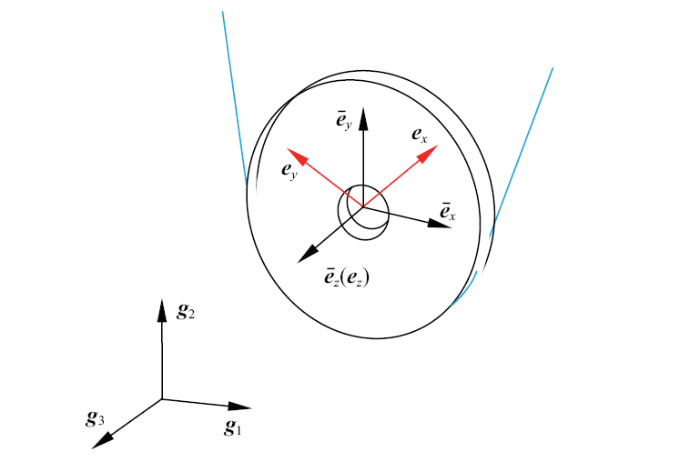Fig.3   The reference and body-fixed coordinate system of a pulley

$\begin{eqnarray}&&{{\bar{ \omega }}}_p = \dot{\beta }_1 {{ g}}_1 + \dot{\beta}_2 {{\bar{ e}}}_y \end{eqnarray}$
$\begin{eqnarray}&&{{\dot{\bar{ \omega }}}}_p = \ddot{\beta }_1 {{ g}}_1 +\ddot{\beta }_2 {{\bar{ e}}}_y +\dot{\beta }_2 \dot{\beta }_1 {{ g}}_1 \times {{\bar{ e}}}_y\end{eqnarray}$

$\begin{eqnarray}&&{{ \omega }}_p = {{\bar{ \omega }}}_p + \dot{\beta }_3 {{ e}}_z \buildrel \Delta \over = {{ T}}_\omega {{\dot{ q}}}_p\end{eqnarray}$
$\begin{eqnarray}&&{{\dot{ \omega }}}_p = {{\dot{\bar{ \omega }}}}_p + \ddot{\beta }_3 {{ e}}_z + \dot{\beta }_3 {{\bar{ \omega }}}_p\times {{ e}}_z \buildrel \Delta \over = {{ T}}_\omega {{\ddot{ q}}}_p + {{ \alpha }}_\omega \end{eqnarray}$

$\begin{eqnarray}&&{{ T}}_\omega = [ {{\begin{array}{*{20}c} { 0_{3\times 3} } \ \ {{{ g}}_1 } \ \ {{{\bar{ e}}}_y } \ \ {{{ e}}_z } \\\end{array} }} ]\end{eqnarray}$

$\begin{eqnarray}{{ \alpha }}_\omega = \dot{\beta }_2 \dot{\beta }_1 {{ g}}_1\times {{\bar{ e}}}_y + \dot{\beta }_3 {{\bar{ \omega }}}_p\times {{ e}}_z\end{eqnarray}$

$\begin{eqnarray}{{ J}}_p = J_p {{ e}}_x {{ e}}_x^{\rm T} + J_p {{ e}}_y {{ e}}_y^{\rm T} + 2J_p {{ e}}_z {{ e}}_z^{\rm T}\end{eqnarray}$

$\begin{eqnarray}\delta w_p = \delta {{\dot{ q}}}^{\rm T}_p ( {{{ M}}_p {{\ddot{ q}}}_p + {{ f}}_p } )\end{eqnarray}$

$\begin{eqnarray}{{ M}}_p = {{ T}}_\omega ^{\rm T} {{ J}}_p {{ T}}_\omega +\lt[ {{\begin{array}{*{20}c} {m{{ E}}_{3\times 3} } \ \ { 0_{3\times 3} } \\ { 0_{3\times 3} } \ \ { 0_{3\times 3} } \\\end{array} }} ]\end{eqnarray}$

$\begin{eqnarray}{{ f}}_p = {{ T}}_\omega ^{\rm T} ( {{{ J}}_p {{ \alpha }}_\omega + {{ \tilde {\omega }}}_p {{ J}}_p {{ \omega }}_p } )\end{eqnarray}$

## 5 滑轮内绳索单元

### 图4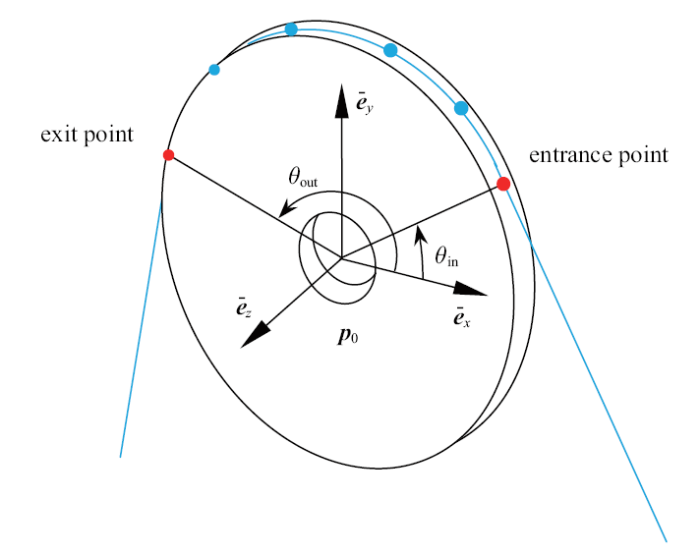Fig.4   A rope element within a pulley

$\begin{eqnarray}{{ r}}_\theta = {{ p}}_0 + r( {\cos \theta {{\bar{ e}}}_x + \sin \theta {{\bar{ e}}}_y } ) \buildrel \Delta\over = {{ p}}_0 + r{{ e}}_n\end{eqnarray}$

$\begin{eqnarray}&&{{\dot{ r}}}_\theta = {{\dot{ p}}}_0 + r{{\bar{ \omega }}}_p \times {{ e}}_n\end{eqnarray}$
$\begin{eqnarray}&&{\partial {{ r}}_\theta }/{\partial t} = {{\dot{ r}}} - (1 + \varepsilon )( {\cos \theta{{\bar{ e}}}_y - \sin \theta {{\bar{ e}}}_x }){\partial s}/{\partial t}\end{eqnarray}$

$\begin{eqnarray}\varepsilon _\theta = r( {s}' )^{ - 1} - 1\end{eqnarray}$

$\begin{eqnarray}&&{\partial s}/{\partial t} = - {s}'\dot{\beta }_3\end{eqnarray}$
$\begin{eqnarray}&&{\partial {s}'}/{\partial t} = - {s}''\dot{\beta }_3\end{eqnarray}$

$\begin{eqnarray}&&\dot{\varepsilon }_\theta = r( {s}' )^{ - 2}{s}''\dot{\beta }_3\end{eqnarray}$
$\begin{eqnarray}&&&{\varepsilon }'_\theta = - r( {s}' )^{ - 3}{s}''\end{eqnarray}$

$\begin{eqnarray}{\partial \varepsilon _\theta }/{\partial t = \dot{\varepsilon }_\theta -}{\varepsilon }'_\theta ( {{\partial s}/{\partial t}} ) = 0\end{eqnarray}$

$\begin{eqnarray}{{\ddot{ r}}}_\theta = {{\ddot{ p}}}_0 + r{{\dot{\bar{ \omega }}}}_p \times {{ e}}_n +r{{\bar{ \omega }}}_p \times ( {{{\bar{ \omega }}}_p \times {{ e}}_n } )\end{eqnarray}$

$\begin{eqnarray}&&{{ {r}'}}_\theta = r( {s}' )^{ - 1}{{ e}}_z \times{{ e}}_n\end{eqnarray}$
$\begin{eqnarray}&&{{ {r}''}}_\theta = - r( {s}' )^{ - 2}{{ e}}_n -r( {s}' )^{ - 3}{s}''{{ e}}_z \times {{ e}}_n\end{eqnarray}$

$\begin{eqnarray}{\partial ^2{{ r}}_\theta }/{\partial t^2} = {{\ddot{ r}}}_\theta - 2{{\dot{ {r}'}}}_\theta \dot{s} + {{ {r}''}}_\theta {\kern 1pt} \dot{s}^2 -{{ {r}'}}_\theta {\kern 1pt} \ddot{s}\end{eqnarray}$

$\begin{eqnarray}&&{{\dot{ {r}'}}}_\theta = r( {s}' )^{ - 1}{{\bar{ \omega }}}_p \times ( {{{ e}}_z \times {{ e}}_n })+r( {s}' )^{ - 2}{s}''\dot{\beta }_3 {{ e}}_z \times {{ e}}_n\end{eqnarray}$
$\begin{eqnarray}&&\ddot{s} = {s}''\dot{\beta }_3^2 - {s}'\ddot{\beta }_3\end{eqnarray}$

$\begin{eqnarray}{\partial ^2{{ r}}_\theta }/{\partial t^2} = {{\ddot{ p}}}_0 + r{{\dot{ \omega }}}_p\times {{ e}}_n +r{{ \omega }}_p \times ( {{{ \omega }}_p \times {{ e}}_n } )\end{eqnarray}$

$\begin{eqnarray}{\partial {{ r}}_\theta }/{\partial t} = [ {{{ E}}_{3\times 3}, - r{{ e}}_n \times[ {{{ g}}_1,{{\bar{ e}}}_y,{{ e}}_z } ]}]{{\dot{ q}}}_p \buildrel \Delta \over = {{ \varGamma }}_r{{\dot{ q}}}_p\end{eqnarray}$

$\begin{eqnarray}\delta p_m = \delta {{\dot{ q}}}_p^{\rm T} ( {{{ M}}_m {{\ddot{ q}}}_p + {{ f}}_m } )\end{eqnarray}$

$\begin{eqnarray}{{ M}}_m = \varphi \int_0^1 {{{ \varGamma }}_r^{\rm T} {{ \varGamma }}_r \rho {s}'{\rm d}\xi }\end{eqnarray}$

$\begin{eqnarray}{{ f}}_m = \varphi \int_0^1 {{{ \varGamma }}_r^{\rm T} {{\dot{ \varGamma }}}_r {{\dot{ q}}}_p \rho {s}'{\rm d}\xi }\end{eqnarray}$

$\begin{eqnarray}&&s = N_1 s_{\rm in} + N_2 s_{\rm out} + r\varphi [N_3 (1 + \varepsilon _{\rm in} )^{ - 1} +\\&&\qquad N_3 (1 + \varepsilon _{\rm out} )^{ - 1}]\end{eqnarray}$

$\begin{eqnarray}&&{s}' = \varphi ^{-1}({N}'_1 s_{\rm in} + {N}'_2 s_{\rm out} ) + r[{N}'_3 (1 + \varepsilon _{\rm in} )^{ - 1} +\\&&\qquad {N}'_4 (1 + \varepsilon _{\rm out} )^{ - 1}]\end{eqnarray}$

## 6 滑轮绳索间边界条件

$\begin{eqnarray}{{ r}}_{\rm in} = {{ p}}_0 + r{{ n}}_{\rm in}\end{eqnarray}$

$\begin{eqnarray}&&{{ n}}_{\rm in} = \cos \theta _{\rm in} {{\bar{ e}}}_x + \sin \theta_{\rm in} {{\bar{ e}}}_y\end{eqnarray}$
$\begin{eqnarray}&&{{ t}}_{\rm in} = \cos \theta _{\rm in} {{\bar{ e}}}_y - \sin \theta_{\rm in} {{\bar{ e}}}_x\end{eqnarray}$

$\begin{eqnarray}{{ {r}'}}_{\rm in} = ( {1 + \varepsilon _{\rm in} } )( {\cos\phi _{\rm in} {{ t}}_{\rm in} + \sin \phi _{\rm in} {{\bar{ e}}}_z } )\end{eqnarray}$

$\begin{eqnarray}{\partial {{ r}}_{\rm in} }/{\partial t = }{{\dot{ p}}}_0 + r{{\bar{ \omega }}}_p \times{{ n}}_{\rm in} + r\dot{\theta }_{\rm in} {{ t}}_{\rm in} - {{ {r}'}}_{\rm in} \dot{s}_{\rm in}\end{eqnarray}$

$\begin{eqnarray}&&r( {\dot{\theta }_{\rm in} - \dot{\beta }_3 } ) = \cos \phi_{\rm in} ( {1 + \varepsilon _{\rm in} } )\dot{s}_{\rm in}\end{eqnarray}$
$\begin{eqnarray}&&r( {\dot{\theta }_{\rm out} - \dot{\beta }_3 } ) = \cos \phi_{\rm out} ( {1 + \varepsilon _{\rm out} } )\dot{s}_{\rm out}\end{eqnarray}$

## 7 卷筒绳索间边界条件

$\begin{eqnarray}{{ r}}_{\rm in} = {{ w}}_0 + z_{\rm in} {{ g}}_z + \bar{r}{{ n}}_{\rm in}\end{eqnarray}$

$\begin{eqnarray}{{ n}}_{\rm in} = \cos \theta _{\rm in} {{ g}}_x + \sin \theta _{\rm in}{{ g}}_y\end{eqnarray}$

$\begin{eqnarray}{{ {r}'}}_{\rm in} = ( {1 + \varepsilon _{\rm in} } )( {\cos \phi _{\rm in} {{ g}}_z \times {{ n}}_{\rm in} + \sin \phi _{\rm in}{{ g}}_z } )\end{eqnarray}$

### 图5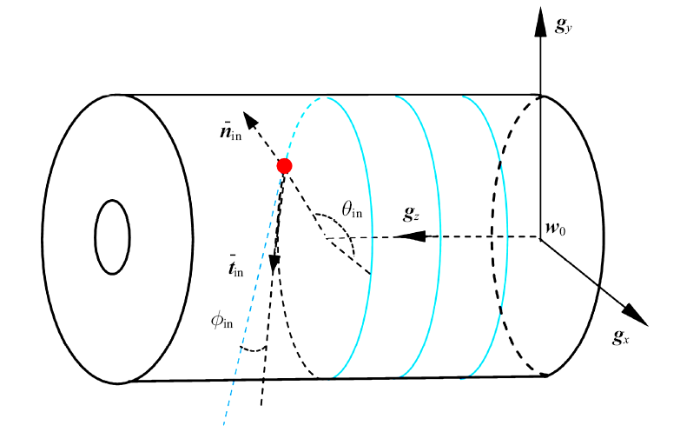Fig.5   The entrance point on a winch

### 7.1 卷筒上无刻槽时

$\begin{eqnarray}{\partial {{ r}}_{\rm in} }/ {\partial t} = {{\dot{ r}}}_{\rm in} - \dot{s}_{\rm in} {{ {r}'}}_{\rm in} =\omega _w \bar{r}{{ g}}_z \times {{ n}}_{\rm in}\end{eqnarray}$

$\begin{eqnarray}&&\bar{r}( {\dot{\theta }_{\rm in} - \omega _w } ) = \dot{s}_{\rm in}( {1 + \varepsilon _{\rm in} } )\cos \phi _{\rm in}\end{eqnarray}$
$\begin{eqnarray}&&\dot{z}_{\rm in} = \dot{s}_{\rm in} ( {1 + \varepsilon _{\rm in} } )\sin\phi _{\rm in}\end{eqnarray}$

### 7.2 卷筒有刻槽时

$\begin{eqnarray}&&{{ r}}_{\rm in} = \bar{r}\cos ( {\gamma _{\rm in} + \vartheta }){{ g}}_x + \bar{r}\sin ( {\gamma _{\rm in} + \vartheta }){{ g}}_y +\\&&\qquad \lambda _z \gamma _{\rm in} {{ g}}_z\end{eqnarray}$
$\begin{eqnarray}&&{{\dot{ r}}}_{\rm in} = {{ g}}_z \times ( {{{ r}}_{\rm in} - \lambda _z \gamma _{\rm in} {{ g}}_z } )( {\dot{\gamma }_{\rm in} + \omega _w } ) + \lambda _z \dot{\gamma }_{\rm in} {{ g}}_z\quad\end{eqnarray}$

$\begin{eqnarray}\vartheta = \int_0^t {\omega _w {\rm d}t}\end{eqnarray}$

$\begin{eqnarray}&&z_{\rm in} = \lambda _z \gamma _{\rm in}\end{eqnarray}$
$\begin{eqnarray}&&\dot{\theta }_{\rm in} = \omega _w + \dot{\gamma }_{\rm in}\end{eqnarray}$

$\begin{eqnarray}{{\bar{ t}}}_{{\rm in}} = \lambda _w^{ - 1} ( {\bar{r}\cos\theta _{\rm in} {{ g}}_y - \bar{r}\sin \theta _{\rm in} {{ g}}_x +\lambda _z {{ g}}_z } )\end{eqnarray}$

$\begin{eqnarray}\lambda _w^2 \,\dot{\gamma }_{\rm in} =(1+ \varepsilon _{\rm in} )\dot{s}_{\rm in}( {\bar{r}\cos \varphi _{\rm in} + \lambda _z \sin \phi _{\rm in} })\end{eqnarray}$

## 8 算例

$$\begin{eqnarray*}v_T = v\end{eqnarray*}$$

$$\begin{eqnarray*}v_{\rm out} = v_T = v\end{eqnarray*}$$

$$\begin{eqnarray*}v_{{\rm in}} = 0\end{eqnarray*}$$

$$\begin{eqnarray*}v_p = \frac12v\end{eqnarray*}$$

$$\begin{eqnarray*}\omega =\frac{v}{2r}\end{eqnarray*}$$

### 图6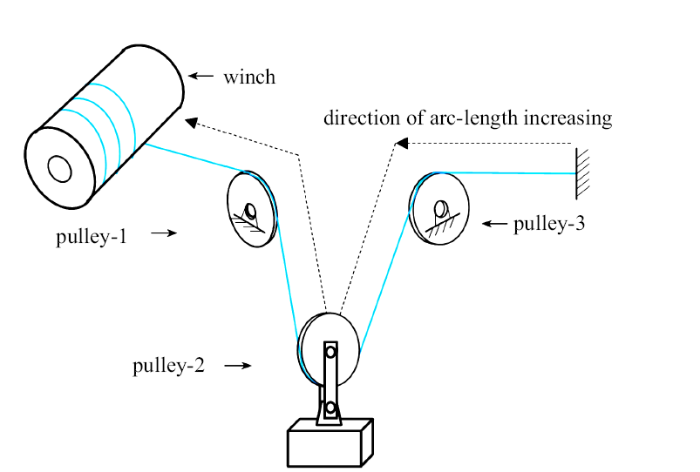Fig.6   A spatial rope-pulley system

Table 1  Origins of coordinate systems for the winch and pulleys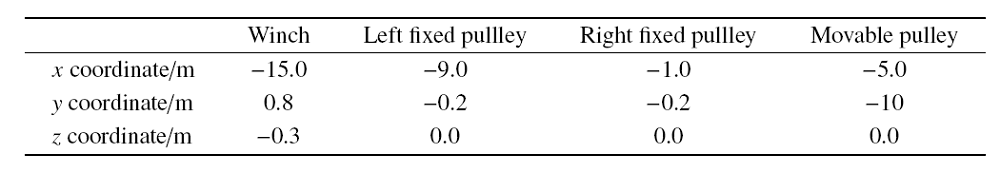Table 2  Initially arclengths along with azimuth and swing angles of the rope at entrance and exit points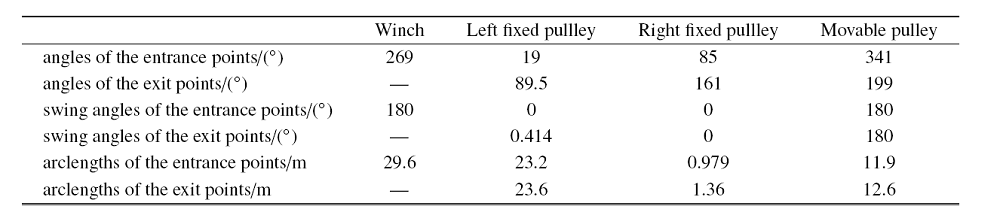### 图7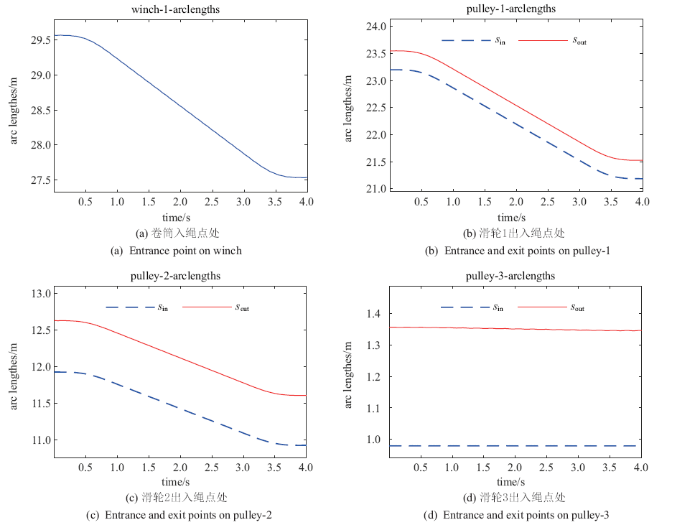Fig.7   Arclengths

### 图8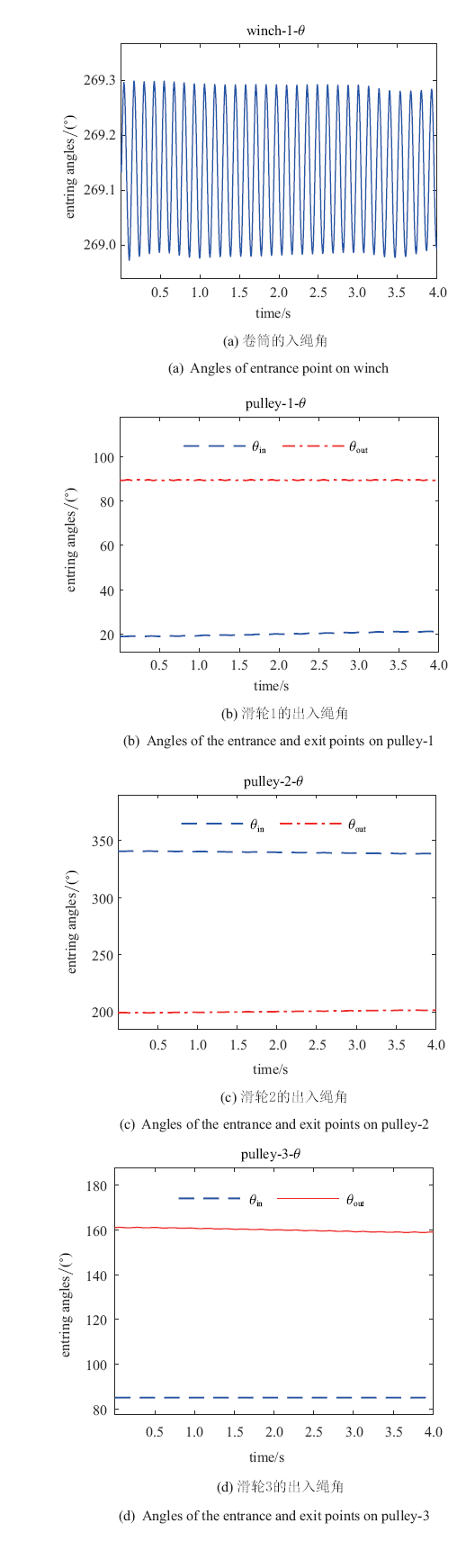Fig.8   Angles of the entrance and exit points

### 图9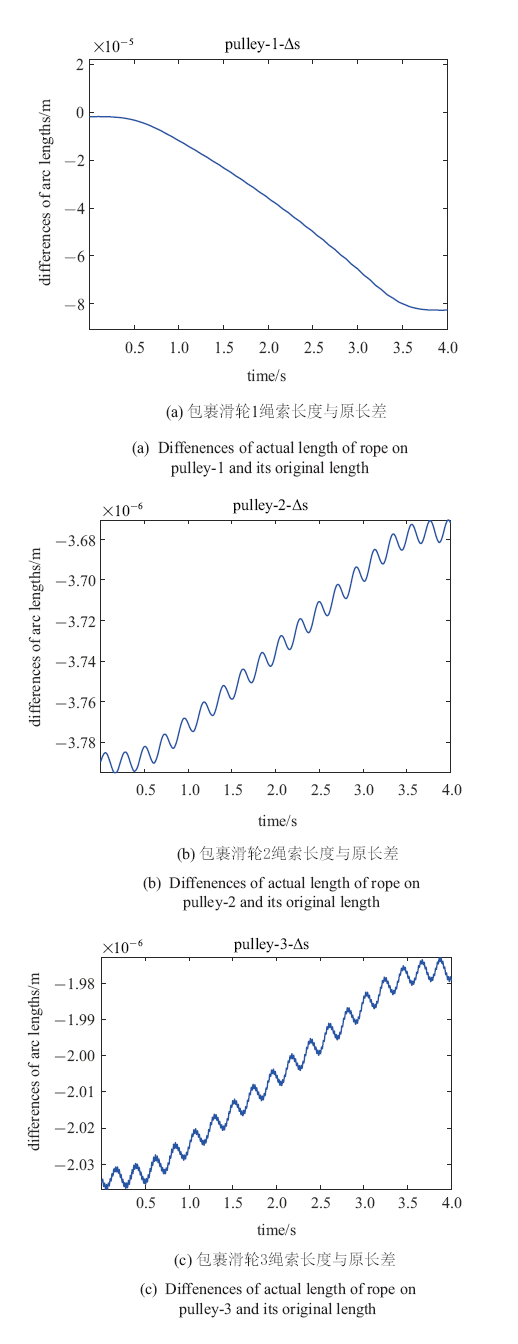Fig.9   Differences of actual length of rope and its original length

### 图10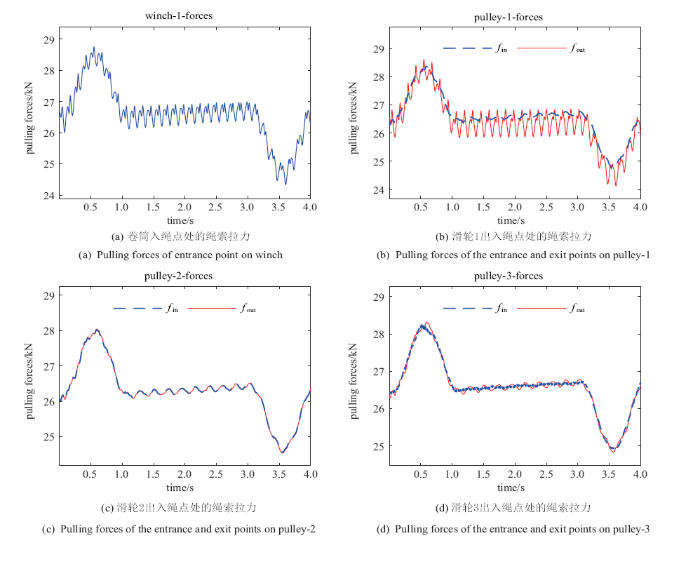Fig.10   Pulling forces

### 图11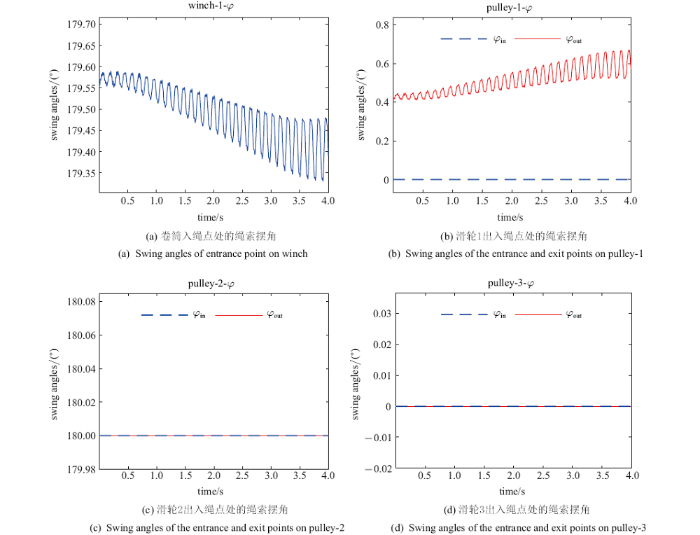Fig.11   Swing angles of the entrance and exit points

### 图12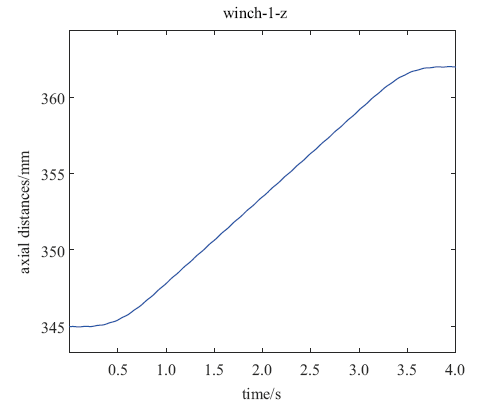Fig.12   Axial coordinates of entrance points on winch

### 图13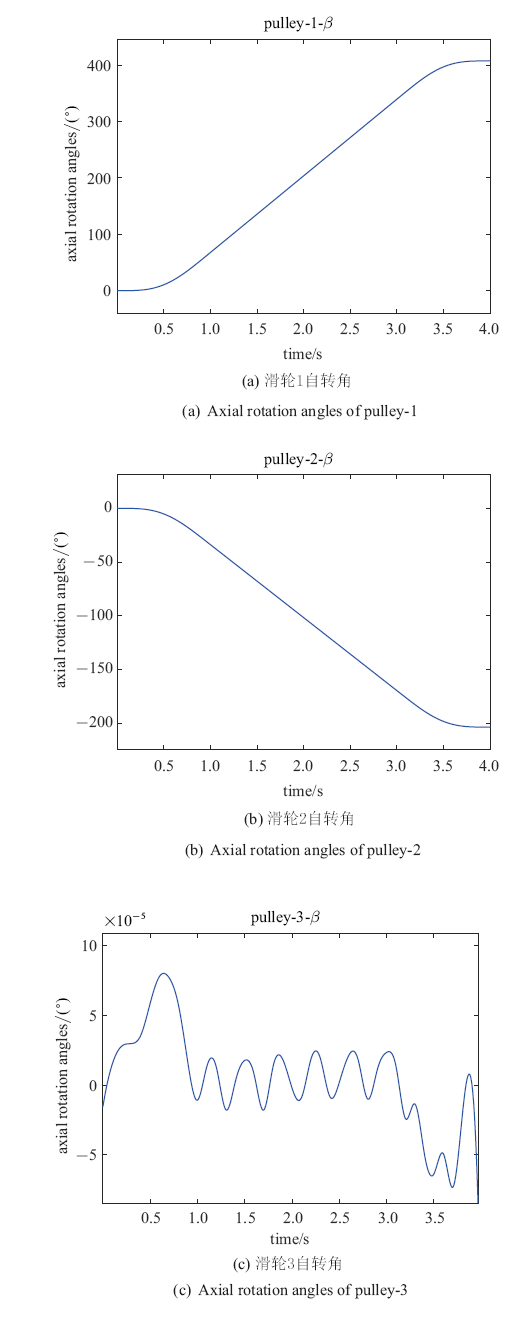Fig.13   Axial rotation angles

### 图14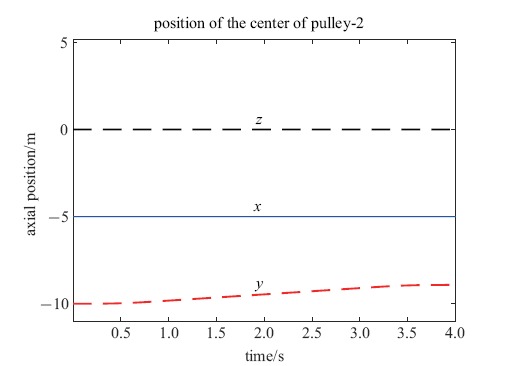Fig.14   Position of the center of the movable pulley

## 9 结论

(1)将不与物质点固定但又有明确物理意义的点作为描述参数, 可有效克服传统方法所引起的困难.

(2)依据虚功率原理可建立复杂系统参数演化方程. 值得特别引起注意的是: 虚功率方程中出现的虚速度是质点物质速度的虚速度, 相应的加速度也应是物质加速度.

(3)空间点的物质速度和加速度可由其空间速度和加速度推演得到, 其表达式中分别含有矢径对弧长的一、二阶导数. 建立节点为空间点的单元时应考虑由此派生出的连续性要求.

(4)按上述原理建立的动态节点绳索单元''适应滑轮绳索系统的特点, 克服了传统方法中滑轮半径严重限制单元尺寸、必须将滑轮绳索间接触力引入系统动力学方程等困难, 能够对滑轮绳索系统进行深入精细的分析.

## 参考文献 原文顺序 文献年度倒序 文中引用次数倒序 被引期刊影响因子

Zienkiewicz OC, Taylor RL, Zhu JZ .

The Finite Element Method: Its Basis and Fundamentals

Elsevier Science, 2013

URL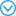The reduction of esters and carboxylic acids to alcohols is a highly relevant conversion for the pharmaceutical and fine-chemical industries and for biomass conversion. It is commonly performed using stoichiometric reagents, and the catalytic hydrogenation of the acids previously required precious metals. Here we report the homogeneously catalyzed hydrogenation of carboxylic acids to alcohols using earth-abundant cobalt. This system, which pairs Co(BF4)2·6H2O with a tridentate phosphine ligand, can reduce a wide range of esters and carboxylic acids under relatively mild conditions (100°C, 80 bar H2) and reaches turnover numbers of up to 8000.

Zienkiewicz OC, Taylor RL .

The Finite Element Method for Solid and Structural Mechanics

Elsevier Science, 2013

URLThe reduction of esters and carboxylic acids to alcohols is a highly relevant conversion for the pharmaceutical and fine-chemical industries and for biomass conversion. It is commonly performed using stoichiometric reagents, and the catalytic hydrogenation of the acids previously required precious metals. Here we report the homogeneously catalyzed hydrogenation of carboxylic acids to alcohols using earth-abundant cobalt. This system, which pairs Co(BF4)2·6H2O with a tridentate phosphine ligand, can reduce a wide range of esters and carboxylic acids under relatively mild conditions (100°C, 80 bar H2) and reaches turnover numbers of up to 8000.

Zienkiewicz OC, Taylor RL, Nithiarasu P .

The Finite Element Method for Fluid Dynamics

Elsevier Science, 2013

URLThe reduction of esters and carboxylic acids to alcohols is a highly relevant conversion for the pharmaceutical and fine-chemical industries and for biomass conversion. It is commonly performed using stoichiometric reagents, and the catalytic hydrogenation of the acids previously required precious metals. Here we report the homogeneously catalyzed hydrogenation of carboxylic acids to alcohols using earth-abundant cobalt. This system, which pairs Co(BF4)2·6H2O with a tridentate phosphine ligand, can reduce a wide range of esters and carboxylic acids under relatively mild conditions (100°C, 80 bar H2) and reaches turnover numbers of up to 8000.

( Qian Weichang. Generalized Variational Principle. Shanghai: Knowledge Publishing House, 1985 (in Chinese))

( Long Yuqiu, Long Zhifei, Cen Song. New Finite Element Theory. Beijing: Tsinghua University Press, 2003 (in Chinese))

Saritas A, Soydas O.

Variational base and solution strategies for non-linear force-based beam finite elements

International Journal of Non-Linear Mechanics, 2012,47(3):54-64

URLThis paper presents the variational bases for the non-linear force-based beam elements. The element state determination of these elements is obtained exactly from a two-field functional with independent stress and strain fields. The variational base of the non-linear force-based beam elements implemented in a general purpose displacement-based finite element program requires the inclusion of independent displacement field in the formulation. For this purpose, a three-field functional is considered with independent displacement, stress, and strain fields. Various local and global solution strategies come out from the mixed formulation of the beam element, and these are shown to yield the algorithms presented for non-linear force formulation beam elements in literature; thus removing any doubts on their variational bases. The presented numerical examples demonstrate the accuracy and robustness of the solution algorithms adapted for mixed formulation elements over popularly used displacement-based beam finite elements even for large structural systems. (C) 2012 Elsevier Ltd.

Lee CK, Angela ML, Hale JS, et al.

Strain smoothing for compressible and nearly-incompressible finite elasticity

Computers & Structures, 2017,182(1):540-555

URLActive development of quantum informational components such as quantum computers and quantum key distribution systems requires parameter characterization of single photon detectors. A key property of the single photon detectors is detection efficiency. One of the methods of the detection efficiency measurement, as listed in the international standard ETSI, is the reference-free twin-photon-based Klyshko method. The signal-to-noise ratio (SNR) of this method depends on the combination of the pump wavelength, the nonlinear crystal's axis angle, and the type of detector's sensitive element. When the combination is difficult, one has to deal with the low SNR of the detector counts measurement. To gain the high SNR, one has to average the long record complicated with the &amp;quot;random telegraph signal&amp;quot; noise. This type of noise exhibits high spectral density at a zero frequency, where simple averaging works. The heterodyne based method we have proposed is to perform averaging at the higher frequency of the modulation introduced to the standard Klyshko measurement scheme. The method was numerically simulated and experimentally tested. The 14 times improvement in SNR for the proposed method relative to the simple averaging was demonstrated by the numerical simulation and confirmed experimentally.

Antonietti P, Verani M, Vergara C, et al.

Numerical solution of fluid-structure interaction problems by means of a high order Discontinuous Galerkin method on polygonal grids

Finite Elements in Analysis & Design, 2019,159(1):1-14

URLThe objectives of this study were to validate a finite element model of the equine distal limb transfixation cast and to determine the effect of six transcortical pin parameters on bone-pin interface (BPI) stresses in the third metacarpal bone.

Sun YP, Chen SC, Yang YQ.

The families of nonconforming mixed finite elements for linear elasticity on simplex grids

Applied Mathematics and Computation, 2019,358(1):348-362

Li YH, Niu RP, Liu GR.

Highly accurate smoothed finite element methods based on simplified eight-noded hexahedron elements

Engineering Analysis with Boundary Elements, 2019,105(1):165-177

Durand R, Pantoja-Rosero BG, Oliveira V.

A general mesh smoothing method for finite elements

Finite Elements in Analysis & Design, 2019,158(1):17-30

URLThe objectives of this study were to validate a finite element model of the equine distal limb transfixation cast and to determine the effect of six transcortical pin parameters on bone-pin interface (BPI) stresses in the third metacarpal bone.

( He Tao,

A partitioned strong coupling algorithm for fluid-structure interaction using arbitrary lagrangian-eulerian finite element formulation

Chinese Journal of Theoretical and Applied Mechanics, 2018,50(2):395-404 (in Chinese))

( Jiang Shouyan, Li Yun, Du Chengbin,

Improved extended scaled boundary finite element methods

Chinese Journal of Theoretical and Applied Mechanics, 2019,51(1):278-288 (in Chinese))

Murdoch AI. Physical Foundations of Continuum Mechanics. Cambridge: Cambridge University Press, 2012

URL( Jin Dongping, Wen Hao, Hu Haiyan,

Modeling,dynamics and control of cable systems

Advances in Mechanics, 2004,34(3):304-313 (in Chinese))

URLURL( Hu Haiyan, Tian Qiang, Zhang Wei, et al.

Nonlinear dynamics and control of large deployable space structures composed of trusses and meshes

Advances in Mechanics, 2013,43(4):390-414 (in Chinese))

URLURL( Pu Hanjun, Xie Xiaopeng, Niu Gaochan, et al.

China Mechanical Engineering, 2012,23(24):2926-2929 (in Chinese))

URLURL( Shen Wenhou, Zhao Zhihua, Ren Gexue, et al.

Multi-body dynamic simulation of impact on cross deck pendant

Journal of Vibration and Shock, 2015,34(5):73-77 (in Chinese))

URLWang JJ, Cao GH, Wang YD, et al.

A novel driving strategy for dynamic simulation of hoisting rope with timeivarying length

International Journal of Modeling Simulation & Scientific Computing, 2013,4(3):135009-1350025

URLOne of two empagliflozin phase 3 trials in type 1 diabetes (EASE-3 but not EASE-2) included a lower 2.5 mg dose. A placebo-corrected HbA1c reduction 0.28% was demonstrated without the increased risk of diabetic ketoacidosis observed at higher doses (10 mg and 25 mg). Since only one trial included the lower dose, we aimed to confirm the observed reduction in HbA1c for the 2.5 mg dose by modeling and simulation analyses.

URL( Chen Weihai, Chen Quanzhu, Liu Rongqiang, et al.

Homing algorithm analysis of a cable-driven humanoid-arm manipulator

Journal of Zhejiang University (Engineering Science), 2013,47(2):345-352 (in Chinese))

URL( Tan Chunlin, Wei Cheng. Basic Research of Rope Dynamics. Beijing: Science Press, 2018 (in Chinese))

( Wang Jun, Zhu Yongning, Xu Jian,

Trajectory simulation of self-propelled elastic rods in fluid

Chinese Journal of Theoretical and Applied Mechanics, 2019,51(1):198-208 (in Chinese))

( Qi Zhaohui, Song Huitao, Zhang Zhigang,

Equilibrium paths and load trajectory deflections of nuclear ring cranes

Engineering Mechanics, 2014,31(1):209-217 (in Chinese))

Fritzkowski P, Kaminski H.

Dynamics of a rope modeled as a multi-body system with elastic joints

Computational Mechanics, 2010,46(6):901-909

URLA discrete model of a rope with spiral springs in joints is considered, the aim being to include transverse elasticity of the rope. Elastic characteristic of the springs is derived on the basis of simple geometrical formulas and the classical curvature-bending moment relationship for beams. Lagrange&#8217;s equations of motion are presented and their complexity is discussed from the computational point of view. Numerical experiments are performed for a system with both scleronomic and rheonomic constraints. The influence of the elasticity on behaviour of the model is analyzed. Results validity is examined in terms of basic energy principles.

Kamman JW, Huston RL.

Multibody dynamics modeling of variable length cable systems

Multibody Syst Dyn, 2001,5(3):211-221

URLThis paper presents a procedure for studying the dynamics ofvariable length cable systems. Such systems commonly occur in deploymentand retrieval (pay-out and reel-in) in cable towing systems such as inship and marine applications.

The cable is modeled as a chainand treated as a multibody system. The chain links in turn are modeledas lumped masses. The pay-out/reel-in process is modeled with variablelength links near the towing point.

Danilin AN, Grishanina TV, Shklyarchuk FN, et al.

Dynamics of a space vehicle with elastic deploying tether

Computers & Structures, 1999,72(1-3):141-147

URLActive development of quantum informational components such as quantum computers and quantum key distribution systems requires parameter characterization of single photon detectors. A key property of the single photon detectors is detection efficiency. One of the methods of the detection efficiency measurement, as listed in the international standard ETSI, is the reference-free twin-photon-based Klyshko method. The signal-to-noise ratio (SNR) of this method depends on the combination of the pump wavelength, the nonlinear crystal's axis angle, and the type of detector's sensitive element. When the combination is difficult, one has to deal with the low SNR of the detector counts measurement. To gain the high SNR, one has to average the long record complicated with the &amp;quot;random telegraph signal&amp;quot; noise. This type of noise exhibits high spectral density at a zero frequency, where simple averaging works. The heterodyne based method we have proposed is to perform averaging at the higher frequency of the modulation introduced to the standard Klyshko measurement scheme. The method was numerically simulated and experimentally tested. The 14 times improvement in SNR for the proposed method relative to the simple averaging was demonstrated by the numerical simulation and confirmed experimentally.

Russell JJ, Anderson WJ.

Equilibrium and stability of a whirling rod-mass system

International Journal of Non-Linear Mechanics, 1977,12(2):91-101

Kamman JW, Huston RL.

Dynamics of constrained multibody systems

Journal of Applied Mechanics, 1984,51(4):899-903

URLInverse dynamics is a technique in which measured kinematics and, possibly, external forces are used to calculate net joint torques in a rigid body linked segment model. However, kinematics and forces are usually not consistent due to incorrect modelling assumptions and measurement errors. This is commonly resolved by introducing 'residual forces and torques' which compensate for this problem, but do not exist in reality. In this study a constrained optimization algorithm is proposed that finds the kinematics that are mechanically consistent with measured external forces and mimic the measured kinematics as closely as possible. The algorithm was tested on datasets containing planar kinematics and ground reaction forces obtained during human walking at three velocities (0.8 m/s, 1.25 and 1.8 m/s). Before optimization, the residual force and torque were calculated for a typical example. Both showed substantial values, indicating the necessity of developing a mechanically consistent algorithm. The proposed optimization algorithm converged to a solution in which the residual forces and torques were zero, without changing the ground reaction forces and with only minor changes to the measured kinematics. When using a rigid body approach, our algorithm ensures a consistent description of forces and kinematics, thereby improving the validity of calculated net joint torque and power values.

URL( Tan Yisong, Liu Yiwei, Liu Hong, et al.

Modeling and capture dynamics of flexible cables used in large-scale space end-effector

Robot, 2011,33(2):156-160 (in Chinese))

URLWittbrodt E, Adamiec-Wójcik I, Wojciech S .

Dynamics of Flexible Multibody Systems: Rigid Finite Element Method

Berlin: Springer, 2006

Ju F, Choo YS.

Super element approach to cable passing through multiple pulleys

International Journal of Solids & Structures, 2005,42(11-12):3533-3547

URLAlthough a resistivity saturation (minimum conductivity) is often observed in disordered metallic solids, such phenomena in the corresponding liquids are not known. Here we report a saturation of the electrical resistivity in Zr_{64}Ni_{36} and Cu_{50}Zr_{50} liquids above a dynamical crossover temperature for the viscosity (T_{A}). The measurements were made for the levitated liquids under the microgravity conditions of the International Space Station. Based on recent molecular dynamics simulations, the saturation is likely due to the ineffectiveness of electron-phonon scattering above T_{A} when the phonon lifetime becomes too short compared to the electron relaxation time. This is different from the conventional resistivity saturation mechanisms in solids.

Hong D, Ren G.

A modeling of sliding joint on one dimensional flexible medium

Multibody Syst Dyn, 2011,26:91-106.

URLThe dynamic modeling of a sliding joint on a one-dimensional medium, such as a cable or a beam, is studied in this paper. The sliding joint is implemented by positioning it at a moving node on the one-dimensional medium, which is realized by variable-length elements at either side of the joint. The variable-length element is established with an absolute nodal coordinate formulation (ANCF) in the framework of the Arbitrary Lagrange-Euler (ALE) description. The sliding of the joint is described by the increasing of the length on one side of the one-dimensional medium and a corresponding decreasing of the other side. In order to capture the discontinuity of the slopes at the position of the sliding joint, the moving node has two slopes as generalized coordinates which are equal to each other in the case of a beam but not in the case of a cable, and in order to avoid the addition-deletion constraint, the node adjacent to the moving node is added or deleted if the element is too long or too short. The governing equations for the coupled system are derived in terms of D'Alembert's principle and the resulting equations of motion are formulated in the standard form of differential algebraic equations of multibody systems. Numerical examples are presented to validate the method proposed by comparing with analytical results which are available or are made possible by simplifying the model.

Escalona JoséL.

An arbitrary Lagrangian--Eulerian discretization method for modeling and simulation of reeving systems in multibody dynamics

Mechanism and Machine Theory, 2017,112:1-21

Sun J, Tian Q, Hu H, et al.

Axially variable-length solid element of absolute nodal coordinate formulation

Acta Mechanica Sinica, 2019,35(3):653-663

Du JL, Bao H, Cui CZ, et al.

Dynamic analysis of cable-driven parallel manipulators with time-varying cable lengths

Finite Elements in Analysis and Design, 2012,48(1):1392-1399

URLIn conventional researches, cables of cable-driven parallel manipulators are treated as simple linear elements that can only work in tension. This results in the fact that the effect of cable dynamics on the positioning precision of the end-effector is not adequately taken into account. To overcome this shortcoming, a dynamic model for cable-driven parallel manipulators with cables of slowly time-varying length is presented in this paper. The partial differential equation characterizing the dynamics of a cable with varying-length is deduced, and converted into ordinary differential equations through spatial discretization by finite difference approximation. Then, the dynamic model for cable-driven parallel manipulators is achieved considering the relationship between the motion of the end-effector and the cable end force, in which the degrees of freedom of cables and the end-effector are all involved. Two numerical examples are demonstrated to validate the dynamic model, and also show that it is necessary to take into consideration the cable dynamics for manipulators of long-span cables. (C) 2011 Elsevier B.V.

Banfi C, Marzocchi A, Musesti A.

On the principle of virtual powers in continuum mechanics

Ricerche Di Matematica, 2006,55(2):139-150

URLWe give a general formulation of the Principle of virtual powers in Continuum Mechanics from a distributional point of view, and study some of its relevant consequences in the field of balance equations.

Keywords: Virtual Powers, Contact Interactions, Balance Equations

Maugin GA.

The principle of virtual power: from eliminating metaphysical forces to providing an efficient modelling tool

Continuum Mechanics & Thermodynamics, 2013,25(2-4):127-146

URLA new therapeutic option to treat osteoporosis is focused on Wnt signaling and its inhibitor sclerostin, a product of the Sost gene. In this work, we study the effect of sclerostin deficiency on trabecular bone formation and resorption in male and female mice and whether it affects mechano-responsiveness. Male and female 10- and 26-week-old Sost knockout (KO) and littermate controls (LCs) were subjected to in vivo mechanical loading of the left tibia for 2 weeks. The right tibia served as internal control. The mice were imaged using in vivo micro-computed tomography at days 0, 5, 10, and 15 and tibiae were collected for histomorphometric analyses after euthanasia. Histomorphometry and micro-CT-based 3D time-lapse morphometry revealed an anabolic and anti-catabolic effect of Sost deficiency although increased trabecular bone resorption accompanied by diminished trabecular bone formation occurred with age. Loading led to diminished resorption in adult female but not in male mice. A net gain in bone volume could be achieved with mechanical loading in Sost KO adult female mice, which occurred through a further reduction in resorbed bone volume. Our data show that sclerostin deficiency has a particularly positive effect in adult female mice. Sclerostin antibodies are approved to treat postmenopausal women with high risk of osteoporotic fractures. Further studies are required to clarify whether both sexes benefit equally from sclerostin inhibition.

( Qi Zhaohui, Cao Yan, Wang Gang,

Model smoothing methods in numerical analysis of flexible multibody systems

Chinese Journal of Theoretical and Applied Mechanics, 2018,50(4):863-870 (in Chinese))

/

 〈〉